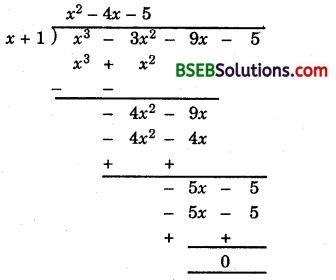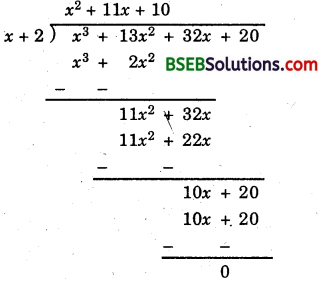# Bihar Board Class 9th Maths Solutions Chapter 2 Polynomials Ex 2.4

Bihar Board Class 9th Maths Solutions Chapter 2 Polynomials Ex 2.4 Textbook Questions and Answers.

## BSEB Bihar Board Class 9th Maths Solutions Chapter 2 Polynomials Ex 2.4Question 1.
Determine which of the following polynomials has (x + 1) a factor:
(i) x³ + x² + x + 1
(ii) x4 + x³ + x² + x + 1
(iii) x4 + 3x³ + 3x² + x + 1
(iv) x³ – x² – (2 + $$\sqrt{2}$$)x + $$\sqrt{2}$$
Solution:
(i) In order to prove that x + 1 is a factor of p(x) = x³ + x² + x + 1, it is sufficient to show that p(- 1) = 0.
Now, p(- 1) = (- 1)³ + (- 1)² + (- 1) + 1
= – 1 + 1 – 1 + 1 = 0
Hence, (x + 1) is a factor of pix) = x³ + x² + x + 1.

(ii) In order to prove that (x + 1) is a factor of
p(x) = x4 + x³ + x² + x + 1, it is sufficient to show that p(- 1) = 0.
Now, p(- 1) = (- 1)4 + (- 1)3 + (- 1)² + (- 1) + 1
= 1 – 1 + 1 – 1 + 1 = 1 ≠ 0
∴ (x + 1) is not a factor of x4 + x³ + x² + x + 1.

(iii) In order to prove that (x + 1) is a factor of
p(x) = x4 + 3x³ + 3x² + x + 1, it is sufficient to show that p(- 1) = 0.
Now, p(- 1) = (- 1)4 + 3(- 1)3 + 3(- 1)² + (- 1) + 1
= 1 – 1 + 1 – 1 + 1 = 1 ≠ 0
∴ (x + 1) is not a factor of x4 + x³ + x² + x + 1.

(iv) In order to prove that (x + 1) is a factor of
p(x) = x³ – x² – (2 + $$\sqrt{2}$$)x + $$\sqrt{2}$$, it is sufficient to show that p(- 1) = 0.
Now, p(- 1) = (- 1)3 – (- 1)² + (2 + $$\sqrt{2}$$)(- 1) + $$\sqrt{2}$$
= 1 – 1 + 2 + $$\sqrt{2}$$ + $$\sqrt{2}$$ = 2$$\sqrt{2}$$ ≠ 0
∴ (x + 1) is not a factor of x³ – x² (2 + $$\sqrt{2}$$)x + $$\sqrt{2}$$.Question 2.
Use the Factor Theorem to determine whether g(x) is a factor of p(x) in each of the following cases :
(i) p(x) = 2x³ + x² – 2x – 1, g(x) = x + 1
(ii) p(x) = x³ + 3x² + 3x + 1, g(x) = x + 2
(iii) p(x) = x³ – 4x² + x + 6, g(x) = x – 3
Solution:
(i) In order to prove that g(x) = x + 1 is a factor of p(x) = 2x³ + x² – 2x – 1, it is sufficient to show that p(- 1) = 0
Now, p(- 1) = 2(- 1)³ + (- 1)² – 2(- 1) – 1
= – 2 + 1 + 2 – 1 = 0
∴ g(x) is a factor of p(x).

(ii) In order to prove that gix) = x + 2 is a factor of p(x) = x³ + 3x² + 3x + 1, it is sufficient to show that p(- 2) = 0
Now, p(- 2) = (- 2)³ + 3(- 2)² – 3(- 2) – 1
= – 8 + 12 – 6 + 1
= – 1 ≠ 0
∴ g(x) is a factor of p(x).

(iii) In order to prove that gix) = x – 3 is a factor of pix) = x³ – 4x² + x – 6, it is sufficient to show that p(+ 3) = 0
Now, p(3) = (3)³ – 4(3)² + 3 – 6
= 27 – 36 + 3 – 6 = 0
= – 12 ≠ 0
∴ g(x) is a factor of p(x).Question 3.
Find the value of k, if x – 1 is a factor of pix) in each of the following cases:
(i) p(x) = x² + x + k
(ii) p(x) = 2x² + kx + $$\sqrt{2}$$
(iii) p(x) = kx² – $$\sqrt{2}$$x + 1
(iv) p(x) = kx² – 3x + k
Solution:
(i) If (x – 1) is a factor of pix) = x² + x + k, then
p(1) = 0
⇒ (1)² + 1 + k = 0
⇒ 1 + 1 + k = 0
⇒ k = – 2

(ii) If (x – 1) is a factor of p(x) = 2x² + kx + $$\sqrt{2}$$, then
p(1). = 0
⇒ 2(1)² + k(1) + $$\sqrt{2}$$ = 0 ⇒ 2 + k + $$\sqrt{2}$$ = 0
⇒ k = – (2 + $$\sqrt{2}$$)

(iii) If (x – 1) is a factor of p(x) = kx² – $$\sqrt{2}$$x + 1, then
p(1) =0
⇒ k(1)² – $$\sqrt{2}$$ (1) + 1 = 0 ⇒ k – $$\sqrt{2}$$ + 1 = 0
⇒ k = $$\sqrt{2}$$ – 1

(iv) If (x – 1) is a factor of p(x) = kx² – 3x + k, then
p(1) = 0
⇒ k(1)² – 3(1) + k = 0 = k – 3 + k = 0
2A = 3 ⇒ k = $$\frac { 3 }{ 2 }$$Question 4.
Factories :
(i) 12x² – 7x + 1
(ii) 2x² + 7x + 3
(iii) 6x² + 5r – 6
(iv) 3x² – x – 4
Solution:
(i) Here p + q = coeff. of x = – 7
pq = coeff.of x² x constant term =
= 12 x 1 = 12
∴ P + q = – 7 = – 4 – 3
and pq = 12 = (- 4)(- 3)
∴ 12x² – 7x + 1 = 12x² – 4x – 3x + 1
= 4x(3x – 1) – 1(3x – 1)
= (3x – 1)(4x – 1)

(ii) Here p + q = coeff. of x = 7
pq = coeff. of x² x constant term
= 2 x 3 = 6
∴ p + q = 7 = 1 + 6
pq = 6 = 1 x 6
∴ 2x² + 7x + 3 = 2x² + x + 6x + 3
= x(2x + 1) + 3(2x + 1)
= (2x + 1)(x + 3)

(iii) Here p + q = coeff. of % = 5
pq = coeff. of x² x constant term
= 6 x – 6 = – 36
p + q = 5 = 9 + (- 4)
and pq = – 36 = 9 x (- 4)
∴ 6x² + 5x – 6 = 6x² + 9x – 4x – 6
= 3x(2x + 3) – 2(2x + 3)
= (2x + 3)(3x – 2)

(iv) Here p + q = coeff. of x = – 1
pq = coeff. of x² x constant term
= 3 x – 4 = – 12
∴ P + q = – 1 = 3 + (- 4)
and pq = – 12 = 3 x (- 4)
∴ 3x² – x – 4 = 3x + 3x – 4x – 4
= 3x(x + 1) – 4(x + 1)
= (x + 1)(3x – 4)Question 5.
Factorise :
(i) x³ – 2x² – x + 2
(ii) x³ – 3x² – 9x – 5
(iii) x³ + 32x² + 32x + 20
(iv) 2y³ + y² – 2y – 1
Solution:
(i) Let f(x) = x³ – 2x² – x + 2
The constant term in fhc) is + 2 and factors of – 2 are ± 1, ± 2.
Putting x = 1 in f(x), we have
f(1) = (1)³ – 2(1)² – 1 + 2
= 1 – 2 – 1 + 2 = 0
∴ (x – 1) is a factor of fix).
Putting x = – 1 in f(x), we have
f(- 1) = (- 1)³ – 2(- 1)² – ( – 1) + 2
= – 1 – 2 + 1 + 2 = o
∴ x + 1 is a factor of f(x).
Putting x = 2 in f(x), we have
f(2) = (2)³ – 2(2)² – (2) + 2
= 8 – 8 – 2 + 2 = 0
∴ (x + 2) is a factor of f(x)
Putting x = – 2 in fix), we have
f(- 2) = (- 2)³ – 2(- 2)² – (- 2) + 2
= – 8 – 8 + 2 + 2
= – 12 ≠ 0
∴ x + 2 is not a factor of fix).
∴ The factors of f(x) are (x – 1), (x + 1) and (x – 2).
Let f(x) = k(x – 1)(x + 1)(x – 2)
⇒ x³ – 2x² – x + 2 = k(x – 1)(x + 1)(x – 2)
Putting x = 0 on both sides, we have
2 = k(- 1)(1)(- 2) ⇒ k = 1
x³ – 2x² – x + 2 = (x – 1)(x + 1)(x – 2)

(ii) Let p(x) = x³ – 3x² – 9x – 5
We shall look for all factors of – 5. These are ± 1, ± 5.
By trial, we find p(- 1) = – 1 – 3 + 9 – 5 = 0. So, (x + 1) is a factor of p(x).∴ p(x) = (x + 1)(x² – 4x – 5)
= (x + 1)(x² + x – 5x – 5)
= (x + 1)[x(x + 1) – 5(x + 1)]
= (x + 1)(x + 1)(x – 5)

(iii) Let p(x) = x³ + 13x² + 32x + 20 .
We shall look for all facotrs of + 20, these are + 1, ± 2, ± 4, ± 5, ± 10 and ± 20.
By trial, we find
p(- 2) = – 8 + 52 – 64 + 20 = 0
∴ (x + 2) is a factor of p(x)
Now, divide p(x) by x + 2.∴ p(x) = (x + 2)(x² + 11x + 10)
= (x + 2)(x² + x + 10x + 10)
= (x + 2)[x(x + 1) + 10(x + 1)]
= (x + 2)(x + 1)(x + 10)

(iv) Let p(y) = 2y³ + y² – 2y – 1
By trial, we find p(1) = 2 + 1 – 2 – 1 = 0.
So, (y – 1) is a factor of p(y).
Now, divide p(y) by y – 1∴ p(y) = (y – 1)(2y² + 3y + 1)
= (y – 1)(2y² + 2y + y + 1)
= (y – 1)[2y(y + 1) + 1(y + 1)]
= (y – 1)(y + 1)(2y + 1)